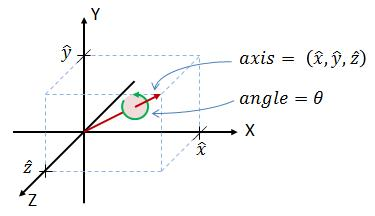# 2 四元数的简单表示

q=q_{0} +iq_{1}+jq_{2}+kq_{3}
\tag{1}

q=\left ( q_{0}, q_{1},q_{2},q_{3} \right )
\tag{2}

# 3 3D旋转的轴角表示\left ( \theta ,\hat{x} ,\hat{y} ,\hat{z} \right )
\tag{3}

# 4 轴角与四元数的相互转换

## 4.1 将轴角转换为四元数

q=q_{0} +iq_{1}+jq_{2}+kq_{3}
\tag{4}

q_{0} = \cos \left ( \frac{\theta }{2} \right )
\tag{5}
q_{1} = \hat{x} \sin \left ( \frac{\theta }{2} \right )
\tag{6}
q_{2} = \hat{y} \sin \left ( \frac{\theta }{2} \right )
\tag{7}
q_{3} = \hat{z} \sin \left ( \frac{\theta }{2} \right )
\tag{8}

q_{0} ^{2} + q_{1} ^{2}+q_{2} ^{2}+q_{3} ^{2} = 1
\tag{9}

## 4.2 将四元数转换为轴角

\theta = 2 \cos ^{-1} \left ( q_{0} \right )
\tag{10}

(\hat{x}, \hat{y}, \hat{z})=\left(\frac{q_{1}}{\sin \left(\frac{\theta}{2}\right)}, \frac{q_{2}}{\sin \left(\frac{\theta}{2}\right)}, \frac{q_{3}}{\sin \left(\frac{\theta}{2}\right)}\right)
\tag{11}

# 5 四元数与旋转矩阵的相互转换

## 5.1 将四元数转换为旋转矩阵

R=\left[\begin{array}{ccc}
q_{0}^{2}+q_{1}^{2}-q_{2}^{2}-q_{3}^{2} & 2 q_{1} q_{2}-2 q_{0} q_{3} & 2 q_{1} q_{3}+2 q_{0} q_{2} \\
2 q_{1} q_{2}+2 q_{0} q_{3} & q_{0}^{2}-q_{1}^{2}+q_{2}^{2}-q_{3}^{2} & 2 q_{2} q_{3}-2 q_{0} q_{1} \\
2 q_{1} q_{3}-2 q_{0} q_{2} & 2 q_{2} q_{3}+2 q_{0} q_{1} & q_{0}^{2}-q_{1}^{2}-q_{2}^{2}+q_{3}^{2}
\end{array}\right]
\tag{12}

R=\left[\begin{array}{lll}
1-2 q_{2}^{2}-2 q_{3}^{2} & 2 q_{1} q_{2}-2 q_{0} q_{3} & 2 q_{1} q_{3}+2 q_{0} q_{2} \\
2 q_{1} q_{2}+2 q_{0} q_{3} & 1-2 q_{1}^{2}-2 q_{3}^{2} & 2 q_{2} q_{3}-2 q_{0} q_{1} \\
2 q_{1} q_{3}-2 q_{0} q_{2} & 2 q_{2} q_{3}+2 q_{0} q_{1} & 1-2 q_{1}^{2}-2 q_{2}^{2}
\end{array}\right]
\tag{13}

## 5.2 将旋转矩阵转换为四元数

R=\begin{bmatrix}
r_{11} & r_{12} & r_{13}\\
r_{21} & r_{22} & r_{23}\\
r_{31} & r_{32} & r_{33}
\end{bmatrix}
\tag{式14}

### 第1步：得到未定义符号的四元数大小

\left | q_{0} \right | = \sqrt{\frac{1+r_{11}+r_{22}+r_{33} }{4} }
\tag{式15}
\left | q_{1} \right | = \sqrt{\frac{1+r_{11}-r_{22}-r_{33} }{4} }
\tag{式16}
\left | q_{2} \right | = \sqrt{\frac{1-r_{11}+r_{22}-r_{33} }{4} }
\tag{式17}
\left | q_{3} \right | = \sqrt{\frac{1-r_{11}-r_{22}+r_{33} }{4} }
\tag{式18}

### 第2步：为了判断正负符号，找到q0,q1,q2,q3的最大值，并且假设其符号为正。然后计算剩余分量，如下所示：

q_{1} = \frac{r_{32}-r_{23} }{4q_{0} }
\tag{式19}
q_{2} = \frac{r_{13}-r_{31} }{4q_{0} }
\tag{式20}
q_{3} = \frac{r_{21}-r_{12} }{4q_{0} }
\tag{式21}

q_{0} = \frac{r_{32}-r_{23} }{4q_{1} }
\tag{式22}
q_{2} = \frac{r_{12}+r_{21} }{4q_{1} }
\tag{式23}
q_{3} = \frac{r_{13}+r_{31} }{4q_{1} }
\tag{式24}

q_{0} = \frac{r_{13}-r_{31} }{4q_{2} }
\tag{式25}
q_{1} = \frac{r_{12}+r_{21} }{4q_{2} }
\tag{式26}
q_{3} = \frac{r_{23}+r_{32} }{4q_{2} }
\tag{式27}

q_{0} = \frac{r_{21}-r_{12} }{4q_{3} }
\tag{式28}
q_{1} = \frac{r_{13}+r_{31} }{4q_{3} }
\tag{式29}
q_{2} = \frac{r_{23}+r_{32} }{4q_{3} }
\tag{式30}